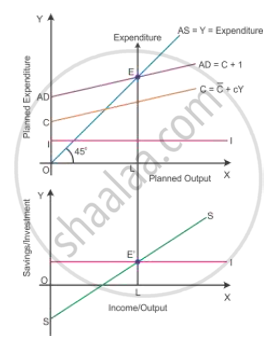# Derive the Two Alternative Conditions of Expressing National Income Equilibrium. Show These Equilibrium Conditions on a Single Diagram. - Economics

Derive the two alternative conditions of expressing national income equilibrium. Show these equilibrium conditions on a single diagram.

#### Solution

Alternative conditions of expressing national income equilibrium:-

1. Aggregate demand and aggregate supply approach (AD and AS approach) Equilibrium level of income is attained only when aggregate demand is equal to aggregate supply. It is the level of output where producers plan to produce the amount of good is equal to consumers plan to purchase the amount of good. Thus, equilibrium is struck where planned output (AS) is equal to planned expenditure (AD) during a period of time.

2. Saving and investment approach (S and I approach)

Equilibrium level of GDP is attained only when planned saving (S) is equal to planned investment (I) during a period of time. Planned investment is what the producers desire to invest according to market expectations.

The equilibrium condition can be diagrammatically explained as follows:-Equilibrium is attained at Point E by using the AD and AS approach in the upper panel of the diagram and at Point E′ by using the S and I approach in the lower panel. Hence, there is only one level of equilibrium output when AS = AD and S = I.

Concept: Concept of Aggregate Demand and Aggregate Supply
Is there an error in this question or solution?